## Sunday, 31 May 2020

### Conceptualising Maths Pre-Session Blog

The most common complaint levied against learning mathematics is that it is parcelled up into discrete methods that have to be memorised and wheeled out when required. What is worse, that also means one has to memorise when every method is required!

Instead, what if we taught what unifies these methods and when it is required? I am talking, of course, about the mathematical concept from which the method arises. I have long advocated that maths teachers should switch their focus from “teaching to do” to “teaching about”. If we teach about mathematical ideas, the structures that underpin them, how these structures interact with each other and how the structure allows us to make sense of how associated procedures do what they do, then learners of mathematics have the ability to recognise when certain procedures are useful as well as how to carry them out. What is more, they are in a position to adapt and improvise for new problems and to select methods appropriate to the situation.

So, what does this look like. Let us take the concept of “perpendicular” as an example. Consider this line segment.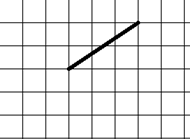What does a line segment that is perpendicular look like? We might choose to have pupils use a protractor to begin with to draw a perpendicular from either end (or not) but we would want pupils to recognise and understand that a perpendicular would have to go two squares right and three squares down (or conversely two squares left and 3 squares). But more importantly we would want pupils to see that this is because the distances that are horizontal become vertical for a perpendicular, and vice versa. Understanding this structure allows pupils much more flexibility to work with perpendiculars. For example, we should now confidently be able to ask pupils about which of these are squares: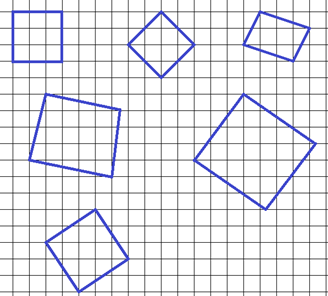Of course, this understanding will eventually be built upon to include perpendicular gradients and perpendicular vectors. But importantly, those are both clearly part of the same structure as this idea. Once 2-D vectors are introduced using the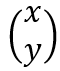notation, it is exactly the same thought process that allows us to see that a perpendicular vector is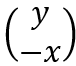, and that another is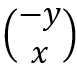. When we understand gradient as the vertical change for a unit horizontal change, we can see that that the perpendicular gradient will become a unit vertical change for a horizontal change that is the negative of the previous vertical change (or, more succinctly, the perpendicular to a gradient of m is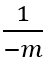). There is no new mathematical structure in the concept of “perpendicular”; the new mathematics is simply how this concept ‘fits’ when we combine it with the concept of “vector” or the concept of “gradient”.

If I could give one piece of advice to any maths teachers (new or experienced) it would be to think about what the mathematical concept is that they want their pupils to learn about, think about all the different concepts they might have to use it with, and then teach it in a way that means it is recognisably the same concept every time pupils encounter it.